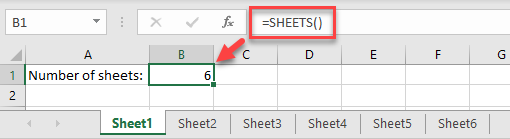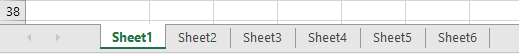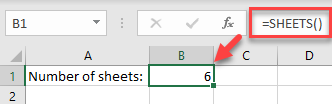# How to Count Number of Worksheets in Excel

This tutorial demonstrates how to count the number of worksheets in an Excel file.## Count Number of Worksheets

The easiest way to count the number of worksheets in your workbook is to use the SHEETS Function. Say your Excel file has six worksheets.In any cell on any of the sheets, enter the formula:

``=SHEETS()``As you can see, this function without arguments returns the total number of sheets in the current workbook (including hidden sheets).

Tips: